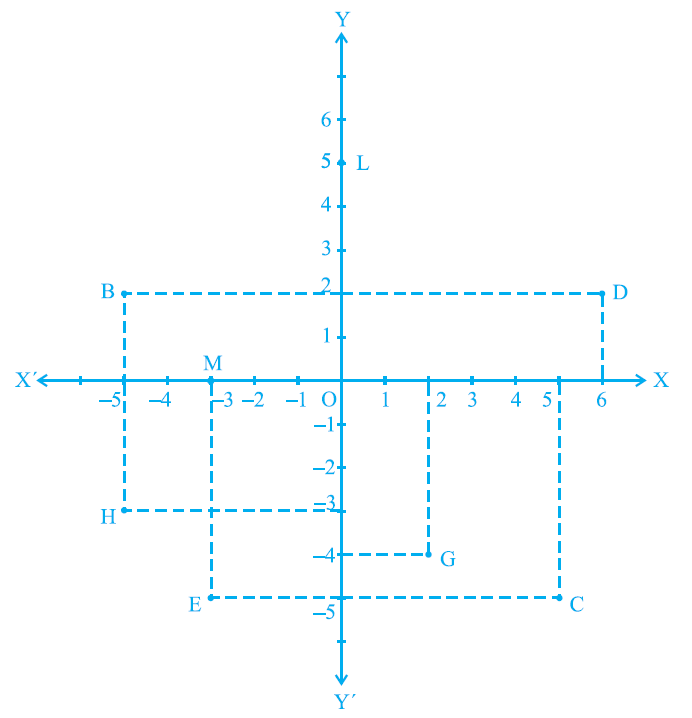# vs.eyeandcontacts.com

## Chapter 3 Coordinate Geometry Exercise 3.2

Question 1: Write the answer of each of the following questions:
i) What is the name of horizontal and the vertical lines drawn to determine the position of any point in the Cartesian plane?
ii) What is the name of each part of the plane formed by these two lines?
iii) Write the name of the point where these two lines intersect.

i) The horizontal line is called the x-axis while the vertical line is known as y-axis.
ii) The quadrant is the name of each part of the plane formed by these two lines.
iii) The origin is the point of intersection of these two lines.

Question 2: See Fig.3.14, and write the following:i) The coordinates of B.
ii) The coordinates of C.
iii) The point identified by the coordinates (–3, –5).
iv) The point identified by the coordinates (2, – 4).
v) The abscissa of the point D.
vi) The ordinate of the point H.
vii) The coordinates of the point L.
viii) The coordinates of the point M.

i) (-5, 2)
ii) (5, -5)
iii) E
iv) G
v) 6
vi) -3
vii) (0, 5)
viii) (-3, 0)